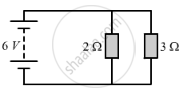Share

# The Figure Given Below Shows an Electric Circuit in Which Current Flows from a 6 V Battery Through Two Resistors. - CBSE Class 10 - Science

#### Question

The figure given below shows an electric circuit in which current flows from a 6 V battery through two resistors.(a) Are the resistors connected in series with each other or in parallel?
(b) For each resistors, state the p.d. across it.
(c) The current flowing from the battery is shared between the two resistors. Which resistor will have bigger share of the current?
(d) Calculate the effective resistance of the tow resistors.
(e) Calculate the current that flows form the battery.

#### Solution

(a) The resistors are connected in parallel.
(b) In a parallel arrangement, the voltage remains the same across each resistor. The battery is also connected in parallel with the resistors. Therefore, the p.d across each resistor is 6 V.
(c) The 2 Ω resistor will have the bigger share of the current since resistance is inversely proportional to the current.
(d) The effective resistance of the parallel combination is:

1R=1R_1+1R_2  1R=12+13 1R=3+26 1R=56R=65ΩR=1.2Ω

1/R=1/R_1+1/R_2

1/R=1/2+1/3

1/R=3+2/6

1/R=5/6

R=6/5  Ω

R=1.2 Ω

(e) The current flowing through the battery, I = 5 A

Is there an error in this question or solution?

#### Video TutorialsVIEW ALL 

Solution The Figure Given Below Shows an Electric Circuit in Which Current Flows from a 6 V Battery Through Two Resistors. Concept: Electric Current and Circuit.
S